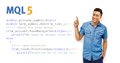# Discussion of article "An Introduction to Fuzzy Logic"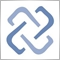Moderator
218609

New article An Introduction to Fuzzy Logic has been published:

Fuzzy logic expands our boundaries of mathematical logic and set theory. This article reveals the basic principles of fuzzy logic as well as describes two fuzzy inference systems using Mamdani-type and Sugeno-type models. The examples provided will describe implementation of fuzzy models based on these two systems using the FuzzyNet library for MQL5.

The mathematical theory of fuzzy sets and fuzzy logic itself originated back in 1965. Its founding father was a Professor Lotfi Zadeh from the University of Berkeley, who first introduced both concepts in his article "Fuzzy Sets" in the Information and Control journal. This mathematical instrument allowed to introduce fuzzy concepts, that anyone could use, to exact science like mathematics, and laid the foundation for fundamentally new methods of problem solving on the basis of soft computing. All these innovations, when utilized properly, can greatly facilitate the process of solving classification problems, creating expert systems as well as building neural networks.

However, the practical application of fuzzy logic did not just stop there, in fact, this mathematical instrument has become predominantly used in automatic control theory. This can be further linked to the emergence of another new concept — fuzzy model, which is a particular case of a mathematical model.

Example:

Let's define a linguistic variable called "Age". By definition, "Age" is a period, a step towards development and growth of a human, animal, plant. The minimum value of this variable is 0, which means that a man is not even a year old. As a maximum value 80 is set up. Depending on the person's age we can give him the following assessment: "newborn", "young", "middle-aged", "old", "senior" etc. This list can accommodate a fairly large number of items. It will be a term set for our linguistic variable and its elements will be terms.

The figure below shows an example of the fuzzy variable "Age", which has only three terms set up: "Young," "Middle-aged,","Old." Each of these terms has its own membership function.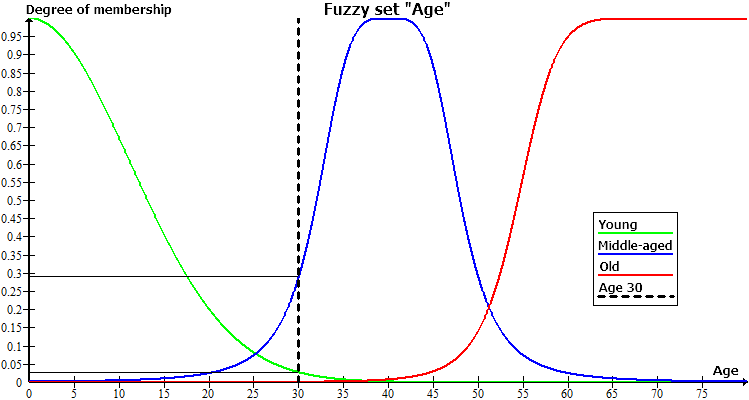Author: MetaQuotes Software Corp.40269

As clearly stated in the title, this is an introduction to fuzzy logic, but that's very rough introduction, don't expect to fully understand it if you don't already know what is fuzzy logic.

The second part is commented code, with as usual examples not related at all to trading.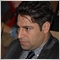15

Hello, I dropt script on chart, and give me this error: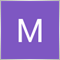34

MetaQuotes:

New article An Introduction to Fuzzy Logic has been published:

Author: MetaQuotes Software Corp.

Hi

when i updated metatrader to build 2342

all of samples with fuzzy logic library

return error "incorrect casting of pointers" on MQL5\Include\Math\Fuzzy\RuleParser.mqh Line 712

many thanks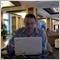Moderator
115792

Hi

when i updated metatrader to build 2342

all of samples with fuzzy logic library

return error "incorrect casting of pointers" on MQL5\Include\Math\Fuzzy\RuleParser.mqh Line 712

many thanks

New MetaTrader 5 Platform Build 2340: Managing account settings in the Tester and expanded integration with Python

Sergey Golubev, 2020.03.02 12:00

If it is related to those codes (Fuzzy - library for developing fuzzy models) so you shoulkd ask the author/coder to update this library (MetaQuotes has nothing to do with it sorry. The website of the author/coder are published in the beginning of this discription: Fuzzy - library for developing fuzzy modelsModerator
115792

hi

when i updated metatrader to build 2342

all of samples with fuzzy logic library

return error "incorrect casting of pointers" on MQL5\Include\Math\Fuzzy\RuleParser.mqh Line 712

many thanks34

Sergey Golubev:

hi

real author is Dmitry Kalyuzhny. but his coded this library in .Net

But maybe someone else changed the code to mql

but I could not find Дмитрий Калюжный profile on mql5

many thanksModerator
115792

hi

real author is Dmitry Kalyuzhny. but his coded this library in .Net

But maybe someone else changed the code to mql

but I could not find Дмитрий Калюжный profile on mql5